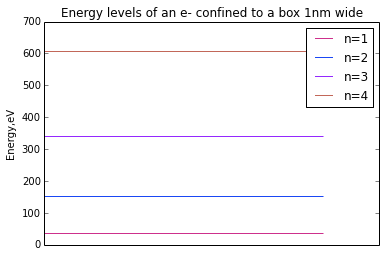# Chapter 3:Wave propoerties of particles¶

## Example no:3.1,Page no:94¶

In :
#Variable declaration
m= 46.0  #mass, gms
v=30.0  #velocity, m/s
h= 6.63*(10**(-34))  #Planck's constant, J.s
m=m/1000.0  #convert to kgs

#Calculation
a=h/(m*v)  #de Broglie wavelength, m
print"The de Broglie wavelength of the golf ball (in m) is:%.2g"%a,"m"
#Part(b)
m= 9.1*(10**(-31))  #mass, kg
v=10**7  #velocity, m/s
h= 6.63*(10**(-34))  #Planck's constant, J.s
a=h/(m*v)  #de Broglie wavelength, mts

#Result
print"de Broglie wavelength for the electron (in m) is:%.2g"%a,"m"

The de Broglie wavelength of the golf ball (in m) is:4.8e-34 m
de Broglie wavelength for the electron (in m) is:7.3e-11 m


## Example no:3.2,Page no:94¶

In :
#Variable declaration
a=10**(-15)  #de Broglie wavelength, mts
Eo= 0.938  #proton rest energy, GeV
h= 4.136*(10**(-15))  #Planck's constant, eV.s
c= 2.998*(10**8)  #velocity of light, m/s

#Calculation
import math
p= h/a  # p is momentum, kg.m/s
pc= (h*c)/a  #Photon's energy, ev
pc=pc*(10**(-9))  #convert to GeV
#pc>E0, relativistic calculation
E= math.sqrt((Eo**2) + (pc**2))  #total energy, GeV
KE = E-Eo  #Kinetic energy, GeV
KE= KE*1000  # convert to MeV

#Result
print"Kinetic Energy of the proton (in MeV) is: ",round(KE),"MeV"

Kinetic Energy of the proton (in MeV) is:  617.0 MeV


## Example no:3.3,Page no:103¶

In :
#Variable declaration
a= 2*(10**(-12))  #de Broglie wavelength, mts
h= 4.136*(10**(-15))  #Planck's constant, eV.s
c= 3*(10**8)  #velocity of light, m/s

#Calculation
pc= (h*c)/a  #p is momentum, pc is electron's energy, eV
pc= pc/1000  #convert to keV
Eo= 511  #rest energy, keV
E= math.sqrt((Eo**2)+(pc**2))  #Total Energy, keV
KE= E-Eo  #Kinetic energy, keV

#Result
print"kinetic energy of the electron (in keV) is,KE: ",round(KE,1),"keV"
vg= c*(math.sqrt(1-(Eo**2/E**2))) #group velocity, m/s
vp= c**2/vg #phase velocity, m/s
print"group velocity of the electron (in m/s) is,vg: ",round(vg/c,3),"c"
print"phase velocity of the electron (in m/s) is,vp:",round(vp/c,1),"c"

kinetic energy of the electron (in keV) is,KE:  292.8 keV
group velocity of the electron (in m/s) is,vg:  0.772 c
phase velocity of the electron (in m/s) is,vp: 1.3 c


## Example no:3.4,Page no:107¶

In :
%matplotlib inline
#Variable declaration
m= 9.1*(10**(-31))  #mass, kg
L= 0.10  #length of box, nm
L= L*(10**(-9))  #convert to m
h= 6.63*(10**(-34))  #Planck's constant, J.s

#Calculation
En=range(4)
p=range(5)
import matplotlib.pyplot as plt
#from numpy import random
import numpy as np
for n in range(1,5):
En[n-1]=(n**2)*(h**2)/(8*m*(L**2))  #Permitted energies, J
print"For level:",n
print"Permitted ernergis :%.2g"%En[n-1],"J"
En[n-1]=38*(n**2)
plt.plot([En[n-1]])
print"Permitted energies (in eV) :",En[n-1], "eV"
p[n]=plt.hlines(En[n-1],0,5, colors=np.random.rand(3,1), linestyles='solid', label='d', hold=None,)
p[n].axes.get_xaxis().set_visible(False)
plt.legend([p,p,p,p],["n=1","n=2","n=3","n=4"])
plt.ylabel("Energy,eV")
plt.title("Energy levels of an e- confined to a box 1nm wide")

plt.show()

#Result
print"\nANS::The minimum energy the electron can have is:",min(En),"n**2 eV\n\n"

Populating the interactive namespace from numpy and matplotlib
For level: 1
Permitted ernergis :6e-18 J
Permitted energies (in eV) : 38 eV
For level: 2
Permitted ernergis :2.4e-17 J
Permitted energies (in eV) : 152 eV
For level: 3
Permitted ernergis :5.4e-17 J
Permitted energies (in eV) : 342 eV
For level: 4
Permitted ernergis :9.7e-17 J
Permitted energies (in eV) : 608 eVANS::The minimum energy the electron can have is: 38 n**2 eV



## Example no:3.5,Page no:107¶

In :
#Variable declaration
m= 10.0  #mass, gms
m= m/1000.0 #convert to kgs
L= 10.0  #Length of box, cms
L= L/100.0 #convert to mts
h= 6.63*(10**(-34)) #Planck's constant, J.s

#Calculation
En=range(5)
for n in range(1,5):
En[n-1]=(n**2)*(h**2)/(8*m*(L**2))  #Permitted energies, J
print"for level:",n
print"Permitted ernergis :%.2g"%En[n-1],"J"

#Result
print "Minimum energy is :En=%.2g"%min(En),"n**2 J,corresponding to n=1"

#corresponding kinetic energy is very low, hence Quantum effects are imperceptible, and Newtonian mechanics is dominant

for level: 1
Permitted ernergis :5.5e-64 J
for level: 2
Permitted ernergis :2.2e-63 J
for level: 3
Permitted ernergis :4.9e-63 J
for level: 4
Permitted ernergis :8.8e-63 J
Minimum energy is :En=5.5e-64 n**2 J,corresponding to n=1


## Example no:3.6,Page no:112¶

In :
#Variable declaration
Xo= 10**(-11)  #uncertainty at time t=o, mts
hb= 1.054*(10**(-34))  #h-bar, reduced Planck's constant, J.s
t= 1  #time, s
m= 1.672*(10**(-27))  #mass, kg

#Calculation
x1= hb*t/(2*m*Xo)  #uncertainty at time t=1, mts

#Result
print"accuracy in position of proton after 1.00 seconds  is ,delta_x>=:%.3g"%x1,"m"

accuracy in position of proton after 1.00 seconds  is ,delta_x>=:3.15e+03 m


## Example no:3.7,Page no:114¶

In :
#Variable declaration
r= 5*(10**(-15))  #radius of nucleus, mts
Xo= 5*(10**(-15))  #assumed initial uncertainty, mts
hb= 1.054*(10**(-34))  #reduced Planck's constant, J.s

#Calculation
p= hb/(2*Xo)  #uncertainty in momentum, kg.m/s
c= 3*(10**8)  #velocity of light, m/s
KE= p*c  #minimum kinetic energy required, J

#Result
print"Uncertainty in momentum of the electron is :%.2g"%p,"kg.m/s"
print"The minimum energy required is :%.2g"%KE,"J \n or"
KE= KE/(1.6*(10**(-19)))  #convert to eV
KE= KE/(10**6)  #convert to MeV
print"The minimum energy required is : ",round(KE),"MeV"


Uncertainty in momentum of the electron is :1.1e-20 kg.m/s
The minimum energy required is :3.2e-12 J
or
The minimum energy required is :  20.0 MeV


## Example no:3.8,Page no:115¶

In :
#Variable declaration
r= 5.3*(10**(-11))  #radius of atom, mts
Xo= 5.3*(10**(-11))  #uncertainty in position, mts
hb= 1.054*(10**(-34))  #Reduced planck Constant, J.s

#Calculation
p= hb/(2*Xo)  #uncertainty in momentum, kg.m/s
m= 9.1*(10**(-31))  #mass, kg
KE= p**2/(2*m)  # minimum kinetic energy, J

#Result
print"The minimum possible kinetic energy for an electron in the atom is :%.2g"%KE,"J"
KE= KE/(1.6*(10**(-19)))  #convert to eV
print"OR,in eV:KE=",round(KE,1),"eV"

The minimum possible kinetic energy for an electron in the atom is :5.4e-19 J
OR,in eV:KE= 3.4 eV


## Example no:3.9,Page no:116¶

In :
#Variable declaration
t= 10**(-8)  #time period between excitation and radiation, s
hb= 1.054*(10**(-34))  #Reduced Planck's constant, J.s

#Calculation
Eo= hb/(2*t)  #uncertainty in photon energy, J
h=hb*(2*(math.pi))  #Planck's constant
Fo= Eo/h  #uncertainty in frequency of light, Hz

#Result
print"Photon energy is uncertain by (in J) :%.2g"%Eo,"J"
print"Frquency of photon is uncertain by (in Hz) :%.2g"%Fo,"Hz"

Photon energy is uncertain by (in J) :5.3e-27 J
Frquency of photon is uncertain by (in Hz) :8e+06 Hz